3.MD.B.3 D Solve one- and two-step how many more - less” problems
 Name:    3.MD.B.3 D Solve one- and two-step how many more - less” problems

Multiple Choice
Identify the choice that best completes the statement or answers the question.

1.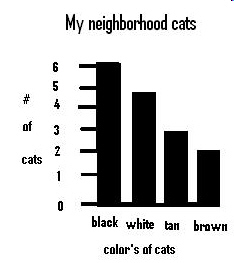According to the graph, how many more black cats are there than tan cats?
 a. 3 c. 9 b. 1 d. 11

2.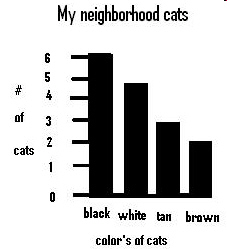According to the graph, each step on the bar is worth how many cats?
 a. 13 c. 2 b. 6 d. 1

3.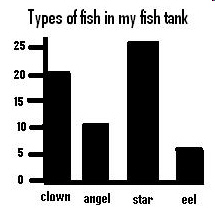According to the graph, how many fewer clown fish are there in the fish tank than star fish?
 a. 0 c. 10 b. 5 d. 45

4.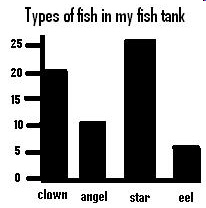ccording to the graph, how many clown fish and star fish are there together?
 a. 45 c. 20 b. 35 d. 25

5.Bob and Tim have the lowest scores of the boys.  Mindy and Nancy have the lowest scores for the girls.  About how much more total score did the girls have than the boys?
 a. 30 c. 20 b. 100 d. 50

6.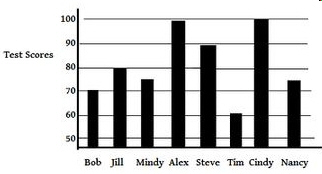About how many less points did Tim score on the test  compared to Cindy?
 a. 20 c. 40 b. 30 d. 50

7.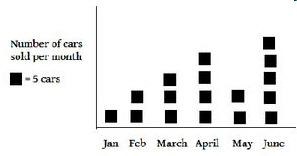How many more cars were sold in June than in January and February?
 a. 15 c. 3 b. 5 d. 10

8.The most cars were sold in April and June.  How many less cars were sold in the other months altogether than in April and June?
 a. 5 c. 50 b. 1 d. 10

9.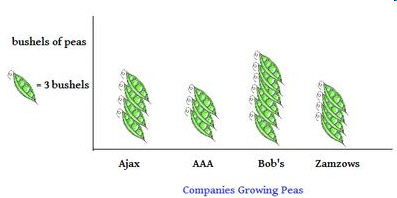How many more bushels of peas did Ajax and AAA companies grow than Bob’s?
 a. 10 c. 7 b. 3 d. 1

10.hich combination of companies below grew the most peas?
 a. Ajax and Zamzows c. Bob’s and AAA b. AAA and Zamzows d. Ajax and Bob’s